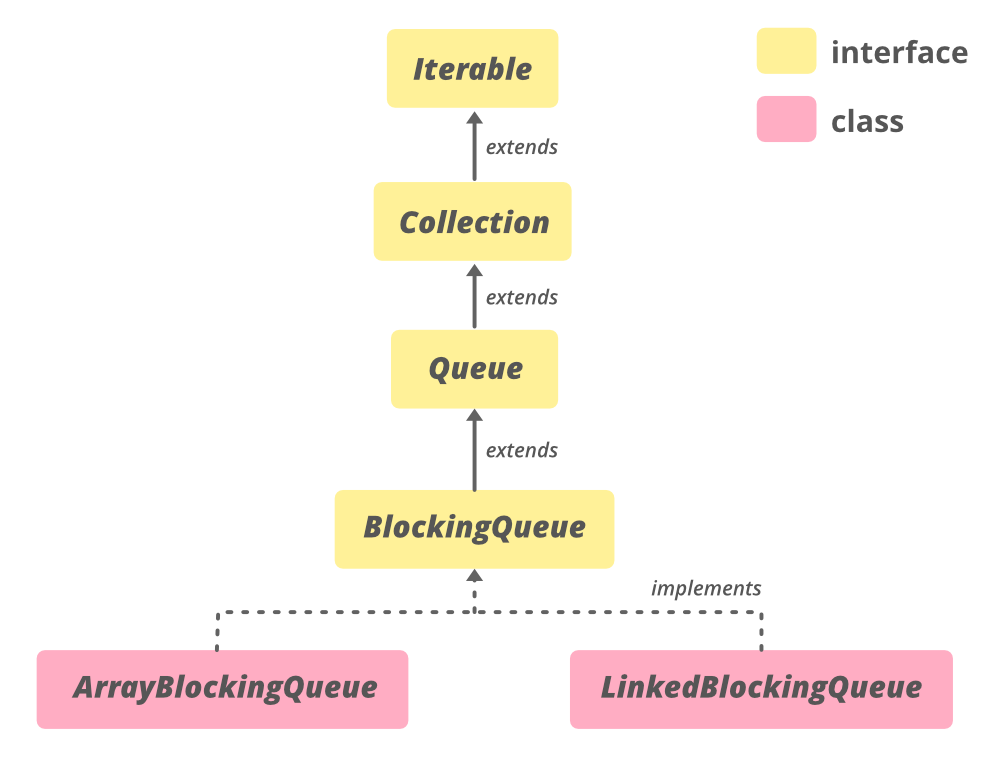# Design Bounded Blocking Queue LeetCode Solution

## Problem Statement

Design Bounded Blocking Queue LeetCode Solution – We need to implement a thread-safe bounded blocking queue that has the following methods:

• Firstly, `BoundedBlockingQueue(int capacity)` The constructor initializes the queue with a maximum `capacity`.
• Secondly, `void enqueue(int element)` Adds an `element` to the front of the queue. If the queue is full, the calling thread is blocked until the queue is no longer full.
• Thirdly, `int dequeue()` Returns the element at the rear of the queue and removes it. If the queue is empty, the calling thread is blocked until the queue is no longer empty.
• Lastly, `int size()` Returns the number of elements currently in the queue.

Your implementation will be tested using multiple threads at the same time. Each thread will either be a producer thread that only makes calls to the `enqueue` method or a consumer thread that only makes calls to the `dequeue` method. The `size` method will be called after every test case.

Here is an example of a Bounded Blocking QueueExample 1:

Input:

```1
1
["BoundedBlockingQueue","enqueue","dequeue","dequeue","enqueue","enqueue","enqueue","enqueue","dequeue"]
[,,[],[],,,,,[]]

```

Output:

```[1,0,2,2]

```

Explanation:

```Number of producer threads = 1
Number of consumer threads = 1

BoundedBlockingQueue queue = new BoundedBlockingQueue(2);   // initialize the queue with capacity = 2.

queue.enqueue(1);   // The producer thread enqueues 1 to the queue.
queue.dequeue();    // The consumer thread calls dequeue and returns 1 from the queue.
queue.dequeue();    // Since the queue is empty, the consumer thread is blocked.
queue.enqueue(0);   // The producer thread enqueues 0 to the queue. The consumer thread is unblocked and returns 0 from the queue.
queue.enqueue(2);   // The producer thread enqueues 2 to the queue.
queue.enqueue(3);   // The producer thread enqueues 3 to the queue.
queue.enqueue(4);   // The producer thread is blocked because the queue's capacity (2) is reached.
queue.dequeue();    // The consumer thread returns 2 from the queue. The producer thread is unblocked and enqueues 4 to the queue.
queue.size();       // 2 elements remaining in the queue. size() is always called at the end of each test case.
```

Example 2:

Input:

```3
4
["BoundedBlockingQueue","enqueue","enqueue","enqueue","dequeue","dequeue","dequeue","enqueue"]
[,,,,[],[],[],]
```

Output:

```[1,0,2,1]

```

Explanation:

```Number of producer threads = 3
Number of consumer threads = 4

BoundedBlockingQueue queue = new BoundedBlockingQueue(3);   // initialize the queue with capacity = 3.

queue.enqueue(1);   // Producer thread P1 enqueues 1 to the queue.
queue.enqueue(0);   // Producer thread P2 enqueues 0 to the queue.
queue.enqueue(2);   // Producer thread P3 enqueues 2 to the queue.
queue.dequeue();    // Consumer thread C1 calls dequeue.
queue.dequeue();    // Consumer thread C2 calls dequeue.
queue.dequeue();    // Consumer thread C3 calls dequeue.
queue.enqueue(3);   // One of the producer threads enqueues 3 to the queue.
queue.size();       // 1 element remaining in the queue.

Since the number of threads for producer/consumer is greater than 1, we do not know how the threads will be scheduled in the operating system, even though the input seems to imply the ordering. Therefore, any of the output [1,0,2] or [1,2,0] or [0,1,2] or [0,2,1] or [2,0,1] or [2,1,0] will be accepted.
```

Constraints:

• `1 <= Number of Prdoucers <= 8`
• `1 <= Number of Consumers <= 8`
• `1 <= size <= 30`
• `0 <= element <= 20`
• The number of calls to `enqueue` is greater than or equal to the number of calls to `dequeue`.
• At most `40` calls will be made to `enque``deque`, and `size`.

## Approach

### Idea:

First of all,`enqueue()` acquires semaphore, and `dequeue()` releases semaphore. Because of this, we need `semaphore` with `permits = capacity` to ensure `queue` does not overflow. Finally, we lock `queue` for critical operations.

## Code

### C++ Program of Design Bounded Blocking Queue:

```class Semaphore {
public:
Semaphore(int permits = 1) : permits(permits) {}

void release() {
lock_guard<mutex> lg(mx);
availablePermits = min(availablePermits + 1, permits);
cv.notify_one();
}

void acquire() {
unique_lock<mutex> ul(mx);
cv.wait(ul, [this]() { return availablePermits > 0; });
availablePermits--;
}

private:
const int permits;
int availablePermits = permits;
mutex mx;
condition_variable cv;
};

class BoundedBlockingQueue {
public:
BoundedBlockingQueue(int capacity) : semaphore(capacity) {}

void enqueue(int element) {
semaphore.acquire();
lock_guard<mutex> lg(mx);
values.push(element);
cv.notify_one();
}

int dequeue() {
unique_lock<mutex> ul(mx);
cv.wait(ul, [this]() { return !values.empty(); });
int element = values.front();
values.pop();
semaphore.release();
return element;
}

int size() {
lock_guard<mutex> lg(mx);
return values.size();
}

private:
queue<int> values;
mutex mx;
condition_variable cv;
Semaphore semaphore;
};```

### Java Program of Design Bounded Blocking Queue:

```class BoundedBlockingQueue {

private final Queue<Integer> queue = new LinkedList<Integer>();
private final Semaphore semaphore;

public BoundedBlockingQueue(int capacity) {
this.semaphore = new Semaphore(capacity);
}

public void enqueue(int element) throws InterruptedException {
semaphore.acquire();
synchronized (queue) {
queue.notify();
}
}

public int dequeue() throws InterruptedException {
int element;
synchronized (queue) {
while (queue.isEmpty()) {
queue.wait();
}
element = queue.poll();
}
semaphore.release();
return element;
}

public int size() {
synchronized (queue) {
return queue.size();
}
}
}```

## Complexity Analysis for Design Bounded Blocking Queue LeetCode Solution:

### Time Complexity

To start off with, the time complexity of the above code is O(1) because we only have a set amount of functions and amount of times they run no matter the input.

### Space Complexity

To end with, the space complexity of the above code is O(1) because since we are only using a set amount of functions and the number of times they run no matter the input, we only use a set amount of space.

Translate »